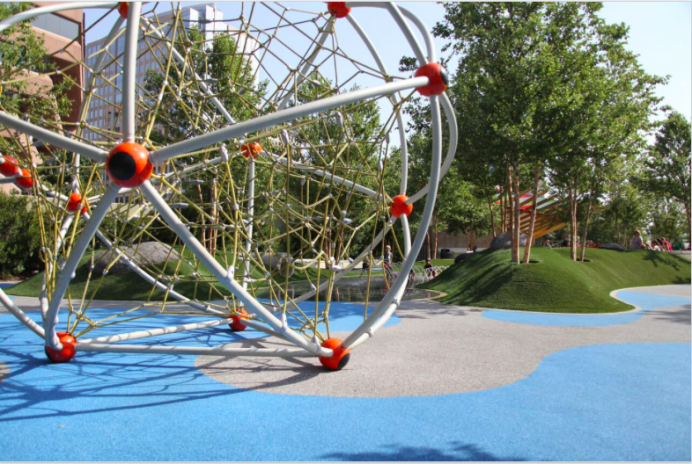# Dallas Arts District: Klyde Warren Park climbing structureIn a park filled with a number of mathematically interesting sights (such as the row of arches along one edge), this climbing structure is arguably the most mathematical object of all. You’ll notice that it is constructed of metal rods joined at the spherical red hubs (with black circles on them). If you look at it further, you will see that exactly five rods meet at every hub, and that every three adjacent hubs are connected in a triangle. So what we have is a structure composed of identical faces, each of which is an regular polygon (in this case, an equilateral triangle), with the same number of faces meeting at every vertex. That’s a very special set of conditions that guarantees that the structure is very symmetric and regular, and it turns out that only five such structures exist, the so-called Platonic solids or regular polyhedra. This particular one is called the regular icosahedron; it has 20 identical triangular faces with five meeting at every vertex. (I recommend passing around a model of the structure.)

### Possible aside: Euler’s formula

If you’re looking for more material, you can count the number of faces (20), bars (what a mathematician calls edges) (30), and hubs (which a mathematician would call vertices) (12). Notice that faces plus vertices differs from edges by only two. If you have models of other polyhedra, participants can compare faces plus vertices with edges, and they will always find the difference is two. This remarkable fact is called Euler’s formula, and it applies to any polyhedron that is roughly speaking ball-shaped (as opposed to doughnut-shaped).

### Symmetry

Above I claimed that this is perhaps the most mathematical object of all in the park, and so I want to show one specific sense in which that’s true. What is the most amount of symmetry that any three-dimensional object can have? Well, it’s the symmetry of the sphere, in which any rotation whatsoever leaves a sphere unchanged. (Good to illustrate this with a ball or other spherical object.) Do you think there’s a second-most amount of symmetry any object can have? It turns out there is. If you look in the center of the climbing structure, amidst the network of ropes to climb on, the centermost cell of the structure is a polyhedron made up entirely of pentagons and hexagons. You might call it a soccer-ball shape, because it is the same as the traditional pattern of panels on a soccer ball; or maybe a chemist would call it a “buckyball” because it is the same shape as a molecule consisting of 60 carbons that goes by that name; but a mathematician would call it a truncated icosahedron. Let’s look into how much symmetry it has. (This is best done with a model.) You can hold it up so that one vertex is at the top and the pentagon that touches that vertex is facing forward. Then have one of the participants choose any other vertex, and show how you can move that vertex to be at the top with the pentagon facing forward. So there is one symmetry for each vertex, and there are 60 vertices. In addition, this polyhedron is mirror-symmetric (looks the same when reflected in a mirror). That doubles the number of symmetries, because any of the rotations can be combined with a reflection.

Thus, this object has 120 different symmetries, which turns out to be the greatest number of symmetries that any object (other than a sphere) can have. Kids get to play in, around, and on it, absorbing its natural mathematical form. (I should mention that the outer structure, the icosahedron, also has 120 symmetries; it’s just much harder to see what they all are and count them with the icosahedron than with the truncated icosahedron,)

### A View to Perot

Speaking of Platonic solids in public places, there’s at least one more of them easily visible from this spot. It’s the Perot Science Center, which is in the shape of a very large cube: identical square faces, with three meeting an each corner (or vertex). You might also ask what the most significant departure is from it being a perfect cube. I think that would have to be the large black structure slanting up the side. Why is it there? It houses escalators to get people to the higher levels of the building. This is another example in which concerns about human accessibility affects the mathematics of the structure.